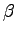# Convergence4

Convergence for Eigenvalue Problems with Mesh Adaptivity

Numerics:

In this example we investigate how our method copes with discontinuous coefficients. We inserted a square subdomain of side 0.5 in the center of the unit square domain. We also choose the functionto be piecewise constant and to assume the value 100 inside the subdomain and the value 1 outside it.

The jump in the value ofcould produce a jump in the gradient of the eigenfunctions all along the boundary of the subdomain. So the regularity of the eigenfunctions in each subdomain, in the sense of Assumption 1.1, is now between. Using uniform refinement, the rate of convergence for eigenvalues should be at leastor equivalently, whereis the number of DOFs. Instead, using our method we obtain greater orders of convergence for big enough value ofand, as can be seen from Table 3. We measure the rate of convergence computing the value ofas before. In fact the rate of convergence fororis close to the rate of convergence for smooth problems. In this case the exact eigenvalueis unknown, but we approximate it by computing the eigenvalue on a very fine mesh involving about half a million of DOFs.

In Figure 3 we depict the mesh coming from the fourth iteration of Algorithm 1 with. This mesh is the result of multiple refinements using both marking strategies 1 and 2 each time. As can be seen the corners of the subdomain are much more refined than the rest of the mesh. This is clearly the effect of the first marking strategy, since the edge residuals have detected the discontinuity in the gradient of the eigenfunction along the interface.

Finally in Figure 4 we depict the eigenfunction corresponding to the smallest eigenvalue of the problem with discontinuous coefficients. This eigenfunction is the one used to refine the mesh in Figure 3.Table 3: Comparison of the reduction of the error and DOFs of the adaptive method for the smallest eigenvalue for the problem with discontinuous coefficients.